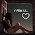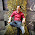Monday, 3 September 2012

CBSE Class 9 - Maths - Ch 7 Triangles (MCQs)

Trianglescredits:openclipart

Q1: In Δ ABC, AB = AC and ∠B = 50°. Then ∠C is equal to

(a) 40°
(b) 50°
(c) 80°
(d) 130°

Q2(CBSE 2010): In ΔABC, ∠C = ∠A and BC = 6 cm and AC = 5 cm. Then the length of AB is:

(a) 6 cm
(b) 5 cm
(c) 3 cm
(d) 2.5 cm

Q3: If one angle of a triangle is equal to the sum of other two angles, then the triangle is

(a) an isosceles triangle
(b) an obtuse triangle
(c) an equilateral triangle
(d) a right triangle

Q4(CBSE 2011): If ΔABC, is right angled at B, then :

(a) AB = AC
(b) AC < AB
(c) AB = BC
(d) AC > AB

Q5(CBSE 2011): In ΔABC if AB = BC, then :

(a) ∠B > ∠C
(b) ∠A = ∠C
(c) ∠A = ∠B
(d) ∠A < ∠B

Q6(CBSE 2010):  In ΔPQR, ∠P = 60°, ∠Q = 50°. Which side of the triangle is the longest ?

(a) PQ
(b) QR
(c) PR
(d) none

Q7(CBSE 2011): P is a point on side BC of  Δ ABC, such that AP bisects ∠BAC, then :

(a) BP = CP
(b) BA > BP
(c) BP > BA
(d) CP < CA

Q8(CBSE 2011):  In the give figure q-8, AD is the median, then ∠BAD  is:
(a) 55°
(b) 50°
(c) 100°
(d) 40°

Q9(CBSE 2010):  If ΔABC ≅ ΔDEF by SSS congruence rule then :

(a) AB = EF, BC = FD, CA = DE
(b) AB = FD, BC = DE, CA = EF
(c) AB = DE, BC = EF, CA = FD
(d) AB = DE, BC = EF, ∠C = ∠F

Q10(CBSE 2011): If  Δ ABC is a right angled at B, then

(a) AB = AC
(b) AC < AB
(c) AB = AC
(d) AC > AB

Q11: In a Δ ABC, ∠A = ∠C. If BC = 3 and  AC = 4 then the perimeter of the triangle is:

(a) 7
(b) 10
(c) 12
(d) 14

Q12: From the following which condition is not possible for the congruence of two triangles ?
(a) ASA
(b) AAS
(c) AAA
(d) SSS

Q13: In a right angled triangle, if one acute angle is half the other, then the smallest angle is:

(a) 15°
(b) 25°
(c) 30°
(d) 35°

1: (b) 50° [Hint: Δ ABC, AB = AC is an isosceles triangle, ∠B =∠C ]

2: (a) 6 cm [ Hint: Δ ABC, ∠C = ∠A, an isosceles triangle, BC = AB]

3: (d) a right triangle
[Hint: Δ ABC, ∠A = ∠B + ∠C, ∵ ∠A + ∠B + ∠C = 180° ⇒ 2∠A = 180° ⇒ ∠A = 90° ]

4: (d) AC > AB [ Hint: Δ ABC, ∠B = 90°, AC is hypotenuse]

5: (b) ∠A = ∠C [Hint: Δ ABC, AB = BC is an isosceles triangle, ∠A =∠C ]

6: (a) PQ [Hint: ∠R = 70° is the largest, the side opposite to the largest angle is the longest.]

7:  (b) BA > BP
[Hint: Consider the triangle as show in fig-q7,
Given, ∠BAP = ∠CAP = z (say)
∠APC is an exterior angle for ΔBAP
⇒ ∠APC = ∠ABP + ∠BAP
⇒ ∠APC > ∠BAP (greater angle has greater side opposite to it.)
⇒ BA > BP]

8: (b) 50°
[Hint:AB = AC ⇒ ∠ABD = ∠ACD = 40°. ⇒ ∠BAC = 100°
Δ ABD ≅  Δ ACD by SSS congruence

9: (c) AB = DE, BC = EF, CA = FD
[Hint: Remember CPCT for corresponding parts of congruent triangles.]

10: (d) AC > AB
[Hint:  ∠B = 90°, AC is hypotenuse the longest side. ]

11: (b) 10 [Hint:  ∠A = ∠C, ⇒AB = BC = 3.]

12: (c) AAA

13: (c) 30° [Hint: x + 2x + 90° = 180° ⇒ 3x = 90°  ⇒ x = 30° ]

.1.good selection of mcq's

2.3.4.5.6.In 7th question (d) can also be right

7.This comment has been removed by the author.